# Part IV: Hidden power breeding in Emerald, Diamond and Pearl

#### Peterko

##### Never give up!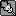PART IV
Hidden power breeding in Emerald, Diamond and Pearl

Other sections of the guide:
Part I: Introduction to breeding

Part II: The Breeding guide

Part III: Emerald, Diamond and Pearl IV breeding combinations probabilities list

Table of content, Part IV:
Section One: Hidden power – general info
1. Type
2. Base power
Section Two: Hidden power breeding in Emerald, Diamond and Pearl

SECTION ONE: Hidden power – general info

Hidden Power is perhaps the most complex move in Pokémon.

The base power of this move ranges from 30 to 70. It can be of any type (except normal), depending on the IV combination that your Pokémon has. In RSFRLGE, it can be either a physical attack or a special attack. It is a physical attack if its type is Fighting, Flying, Poison, Ground, Rock, Bug, Ghost or Steel; it is a special attack if its type is Fire, Water, Grass, Electric, Psychic, Ice, Dragon or Dark. In DP, there is no such complexity, since it is always a special attack.

1. Type

Your Hidden Power type is determined by whether or not your IVs are even or odd. An even IV is an IV which can be divided by two without remainder, otherwise it is an odd IV. Namely:

Even = IV 30, 28, 26, 24, 22, 20, 18, 16, 14, 12, 10, 8, 6, 4, 2, 0
Odd = IV 31, 29, 27, 25, 23, 21, 19, 17, 15, 13, 11, 9, 7, 5, 3, 1

The easiest way to discover the type of your Hidden Power is to start from zero, and:

1) Add 32 if the Special Defense IV is odd.
2) Add 16 if the Special Attack IV is odd.
3) Add 8 if the Speed IV is odd.
4) Add 4 if the Defense IV is odd.
5) Add 2 if the Attack IV is odd.
6) Add 1 if the HP IV is odd.

Depending on the resulting number, the type of your Hidden Power can be found using the following chart:

Between 0 and 4: Fighting
Between 5 and 8: Flying
Between 9 and 12: Poison
Between 13 and 16: Ground
Between 17 and 20: Rock
Between 21 and 25: Bug
Between 26 and 29: Ghost
Between 30 and 33: Steel
Between 34 and 37: Fire
Between 38 and 41: Water
Between 42 and 46: Grass
Between 47 and 50: Electric
Between 51 and 54: Psychic
Between 55 and 58: Ice
Between 59 and 62: Dragon
63: Dark

Let's have an example: Suppose your Pokémon has the following IVs (remember, in the order HP, Attack, Defense, Special Attack, Special Defense, Speed):

11, 8, 23, 22, 31, 4

We start from zero, then add 1 because of an odd HP IV (11), add 4 because of an odd Def IV (23), and add 32 because of an odd SpDef IV (31). The answer is 37, which corresponds to Hidden Power Fire.

2. Base Power

The base power of your Hidden Power can be found as follows.

Start from zero. Then, for each IV, if the IV divided by two is an odd number (rounded down), i.e. IV = 31 or 30 (15), 27 or 26 (13), 23 or 22 (11), 19 or 18 (9), 15 or 14 (7), 11 or 10 (5), 7 or 6 (3), or 3 or 2 (1)

then:

1) Add 32 if the IV is the Special Defense IV.
2) Add 16 if the IV is the Special Attack IV.
3) Add 8 if the IV is the Speed IV.
4) Add 4 if the IV is the Defense IV.
5) Add 2 if the IV is the Attack IV.
6) Add 1 if the IV is the HP IV.

After finishing, multiply this number by 40, then divide the answer by 63, and round down. Finally, add 30 to this answer, and you have your Hidden Power base power.

For example, for the IVs given earlier:

11, 8, 23, 22, 31, 4

We start from zero, then add 1 because the HP IV divided by 2 is odd (11 / 2 = 5 rounded down, which is odd), add 4 because of an the Def IV divided by 2 is odd (23 / 2 = 11 rounded down), add 16 since the SpAtk IV divided by 2 is odd (22 / 2 = 11) and add 32 since the SpDef IV divided by 2 is odd (31 / 2 = 15 rounded down). The answer is 53. Multiplying by 40 gives 2120. Dividing 2120 by 63 and rounding down, we get 33. Finally we add 30 to this, getting 63. So Hidden Power's base power would be 63 in this case.

If you haven't understood a single thing from the above, you don't need to worry, since you don´t have to know how exactly the base power is calculated. The most important thing to know is that if the Special Defense, Special Attack and Speed IVs (all three) are odd numbers after being divided by two, you will always get at least base power 65. Because it is relatively easy to breed 3 IVs, these are the three IVs you should aim for when breeding for your Hidden Power.

SECTION TWO: Hidden power breeding in Emerald, Diamond and Pearl

FORMAT: HP/attack/defense/special attack/special defense/speed

parent A + parent B -> baby = probability in % = one in how many eggs (rounded up)

NOTE: it doesn´t matter which IV you start with, or in which order, because the three main hidden power IVs (sp.defense, sp.attack, speed) have all the same probability to be passed in Emerald (D/P), it would only matter if one of those three IVs had to be HP or defense, but this is not the case...so feel free to start with any of those three IVs

Order: ice, grass, fighting, ground, rock, flying, electric, bug, ghost, fire, water, psychic, dragon, dark, steel, poison (CTRL+F)

hidden power ICE
first way – max speed, special attack and special defense
second way – max special attack and special defense, 30 speed
-> here is only the first way, max speed is more important + it has a higher chance to get the ICE type

1.
x/x/x/x/x/31 + x/x/x/x/x/x -> x/x/x/x/x/31 = 26.5625% = 1:4
2.
x/x/x/x/31/x + x/x/x/x/x/31 -> x/x/x/x/31/31 = 5.95703125% = 1:17
3.
x/x/x/x/31/31 + x/x/x/31/x/x -> x/x/x/31/31/x = 5.95703125% = 1:17, or
x/x/x/x/31/31 + x/x/x/31/x/x -> x/x/x/31/x/31 = 5.95703125% = 1:17
4.
x/x/x/31/31/x + x/x/x/31/x/31 -> x/x/x/31/31/31 = 1.858062744% = 1:54, or
x/x/x/31/31/x + x/x/x/x/31/31 -> x/x/x/31/31/31 = 1.858062744% = 1:54, or
x/x/x/31/x/31 + x/x/x/x/31/31 -> x/x/x/31/31/31 = 1.858062744% = 1:54
5.
x/x/x/31/31/31 + x/x/x/31/31/x -> x/x/x/31/31/31 = 3.474273682% = 1:29, or
x/x/x/31/31/31 + x/x/x/31/x/31 -> x/x/x/31/31/31 = 3.474273682% = 1:29, or
x/x/x/31/31/31 + x/x/x/x/31/31 -> x/x/x/31/31/31 = 3.474273682% = 1:29
6.
x/x/x/31/31/31 + x/x/x/31/31/31 -> x/x/x/31/31/31 = 6.4503479% = 1:16

every approximately 16th baby will now have a chance to have hidden power ICE with base power at least 65, if

A) defense IV and attack IV are even numbers, HP IV is an odd number
B) defense IV and HP IV are even numbers, attack IV is an odd number
C) defense IV, attack IV and HP IV are all even numbers

Even number = IV 30, 28, 26, 24, 22, 20, 18, 16, 14, 12, 10, 8, 6, 4, 2, 0
Odd number = IV 31, 29, 27, 25, 23, 21, 19, 17, 15, 13, 11, 9, 7, 5, 3, 1

For max base power (70) hidden power ICE, besides getting one of the options A), B) or C), you will need the exact IVs of HP, attack and defense to be one of these:
31/30, 27/26, 23/22, 19/18, 15/14, 11/10, 7/6, 3/2

hidden power GRASS
max speed and special defense, 30 special attack

1.
x/x/x/x/x/31 + x/x/x/x/x/x -> x/x/x/x/31 = 26.5625% = 1:4
2.
x/x/x/x/x/31 + x/x/x/x/31/x -> x/x/x/x/31/31 = 5.95703125% = 1:17
3.
x/x/x/x/31/31 + x/x/x/30/x/x -> x/x/x/30/x/31 = 5.95703125% = 1:17, or
x/x/x/x/31/31 + x/x/x/30/x/x -> x/x/x/30/31/x = 5.95703125% = 1:17
4.
x/x/x/30/31/x + x/x/x/30/x/31 -> x/x/x/30/31/31 = 1.858062744% = 1:54, or
x/x/x/30/31/x + x/x/x/x/31/31 -> x/x/x/30/31/31 = 1.858062744% = 1:54, or
x/x/x/30/x/31 + x/x/x/x/31/31 -> x/x/x/30/31/31 = 1.858062744% = 1:54
5.
x/x/x/30/31/31 + x/x/x/30/31/x -> x/x/x/30/31/31 = 3.474273682% = 1:29, or
x/x/x/30/31/31 + x/x/x/x/31/31 -> x/x/x/30/31/31 = 3.474273682% = 1:29, or
x/x/x/30/31/31 + x/x/x/30/x/31 -> x/x/x/30/31/31 = 3.474273682% = 1:29
6.
x/x/x/30/31/31 + x/x/x/30/31/31 -> x/x/x/30/31/31 = 6.4503479% = 1:16

every approximately 16th baby will now have a chance to have hidden power GRASS with base power at least 65, if

A) HP IV is an even number, attack IV and defense IV are odd numbers
B) attack IV is an even number, HP IV and defense IV are odd numbers
C) defense IV is an even number, HP IV and attack IV are odd numbers
D) HP IV and attack IV are even numbers, defense IV is an odd number
E) HP IV and defense IV are even numbers, attack IV is an odd number

Even number = IV 30, 28, 26, 24, 22, 20, 18, 16, 14, 12, 10, 8, 6, 4, 2, 0
Odd number = IV 31, 29, 27, 25, 23, 21, 19, 17, 15, 13, 11, 9, 7, 5, 3, 1

For max base power (70) hidden power GRASS, besides getting one of the options A)-E), you will need the exact IVs of HP, attack and defense to be one of these:
31/30, 27/26, 23/22, 19/18, 15/14, 11/10, 7/6, 3/2

NOTE: if you want to have a chance to get both hidden power ICE and GRASS, one parent should have x/x/x/30/31/31 and the second x/x/x/31/31/31 (they differ only in sp.attack)

hidden power FIGHTING
30 speed, special attack and special defense

1.
x/x/x/x/30/x + x/x/x/x/x/x -> x/x/x/x/30/x = 26.5625% = 1:4
2.
x/x/x/x/30/x + x/x/x/x/x/30 -> x/x/x/x/30/30 = 5.95703125% = 1:17
3.
x/x/x/x/30/30 + x/x/x/30/x/x -> x/x/x/30/30/x = 5.95703125% = 1:17, or
x/x/x/x/30/30 + x/x/x/30/x/x -> x/x/x/30/x/30 = 5.95703125% = 1:17
4.
x/x/x/x/30/30 + x/x/x/30/30/x -> x/x/x/30/30/30 = 1.858062744% = 1:54, or
x/x/x/x/30/30 + x/x/x/30/x/30 -> x/x/x/30/30/30 = 1.858062744% = 1:54, or
x/x/x/30/x/30 + x/x/x/30/30/x -> x/x/x/30/30/30 = 1.858062744% = 1:54
5.
x/x/x/30/30/30 + x/x/x/x/30/30 -> x/x/x/30/30/30 = 3.474273682% = 1:29, or
x/x/x/30/30/30 + x/x/x/30/x/30 -> x/x/x/30/30/30 = 3.474273682% = 1:29, or
x/x/x/30/30/30 + x/x/x/30/30/x -> x/x/x/30/30/30 = 3.474273682% = 1:29
6.
x/x/x/30/30/30 + x/x/x/30/30/30 -> x/x/x/30/30/30 = 6.4503479% = 1:16

every approximately 16th baby will now have a chance to have hidden power FIGHTING with base power at least 65, if

A) defense IV is an even number (HP IV and attack IV don ´t matter)

For max base power (70) hidden power FIGHTING, besides getting even defense, you will need the exact IVs of HP, attack and defense to be one of these:
31/30, 27/26, 23/22, 19/18, 15/14, 11/10, 7/6, 3/2

hidden power GROUND
first way – max speed, 30 special attack and special defense
second way – max special attack, 30 speed and special defense
-> here is only the first way, max speed is more important + it has a higher chance to get the GROUND type

1.
x/x/x/x/30/x + x/x/x/x/x/x -> x/x/x/x/30/x = 26.5625% = 1:4
2.
x/x/x/x/30/x + x/x/x/30/x/x -> x/x/x/30/30/x = 5.95703125% = 1:17
3.
x/x/x/30/30/x + x/x/x/x/x/31 -> x/x/x/30/x/31 = 5.95703125% = 1:17, or
x/x/x/30/30/x + x/x/x/x/x/31 -> x/x/x/x/30/31 = 5.95703125% = 1:17
4.
x/x/x/30/30/x + x/x/x/30/x/31 -> x/x/x/30/30/31 = 1.858062744% = 1:54, or
x/x/x/30/30/x + x/x/x/x/30/31 -> x/x/x/30/30/31 = 1.858062744% = 1:54, or
x/x/x/x/30/31 + x/x/x/30/x/31 -> x/x/x/30/30/31 = 1.858062744% = 1:54
5.
x/x/x/30/30/31 + x/x/x/30/30/x -> x/x/x/30/30/31 = 3.474273682% = 1:29, or
x/x/x/30/30/31 + x/x/x/30/x/31 -> x/x/x/30/30/31 = 3.474273682% = 1:29, or
x/x/x/30/30/31 + x/x/x/x/30/31 -> x/x/x/30/30/31 = 3.474273682% = 1:29
6.
x/x/x/30/30/31 + x/x/x/30/30/31 -> x/x/x/30/30/31 = 6.4503479% = 1:16

every approximately 16th baby will now have a chance to have hidden power GROUND with base power at least 65, if

A) defense IV and attack IV are odd numbers, HP IV is an even number
B) defense IV and HP IV are odd numbers, attack IV is an even number
C) defense IV, HP IV and attack IV are all odd numbers

For max base power (70) hidden power GROUND, besides getting one of the options A)-C), you will need the exact IVs of HP, attack and defense to be one of these:
31/30, 27/26, 23/22, 19/18, 15/14, 11/10, 7/6, 3/2

hidden power ROCK
max special attack, 30 speed and special defense

1.
x/x/x/31/x/x + x/x/x/x/x/x -> x/x/x/31/x/x = 26.5625% = 1:4
2.
x/x/x/31/x/x + x/x/x/x/30/x -> x/x/x/31/30/x = 5.95703125% = 1:17
3.
x/x/x/31/30/x + x/x/x/x/x/30 -> x/x/x/31/x/30 = 5.95703125% = 1:17, or
x/x/x/31/30/x + x/x/x/x/x/30 -> x/x/x/x/30/30 = 5.95703125% = 1:17
4.
x/x/x/31/30/x + x/x/x/31/x/30 -> x/x/x/31/30/30 = 1.858062744% = 1:54, or
x/x/x/31/30/x + x/x/x/x/30/30 -> x/x/x/31/30/30 = 1.858062744% = 1:54, or
x/x/x/x/30/30 + x/x/x/31/x/30 -> x/x/x/31/30/30 = 1.858062744% = 1:54
5.
x/x/x/31/30/30 + x/x/x/31/30/x -> x/x/x/31/30/30 = 3.474273682% = 1:29, or
x/x/x/31/30/30 + x/x/x/31/x/30 -> x/x/x/31/30/30 = 3.474273682% = 1:29, or
x/x/x/31/30/30 + x/x/x/x/30/30 -> x/x/x/31/30/30 = 3.474273682% = 1:29
6.
x/x/x/31/30/30 + x/x/x/31/30/30 -> x/x/x/31/30/30 = 6.4503479% = 1:16

every approximately 16th baby will now have a chance to have hidden power ROCK with base power at least 65, if

A) HP IV is an odd number, attack IV and defense IV are even numbers
B) attack IV is an odd number, HP IV and defense IV are even numbers
C) defense IV is an odd number, HP IV and defense IV are even numbers
D) HP IV and attack IV are odd numbers, defense IV is an even number

For max base power (70) hidden power ROCK, besides getting one of the options A)-D), you will need the exact IVs of HP, attack and defense to be one of these:
31/30, 27/26, 23/22, 19/18, 15/14, 11/10, 7/6, 3/2

hidden power FLYING
first way – max speed, 30 special attack and special defense
second way – 30 speed, special attack and special defense
here are both, the first one has max speed but the second has a much higher chance to get the FLYING type

first way
1.
x/x/x/x/x/31 + x/x/x/x/x/x -> x/x/x/x/x/31 = 26.5625% = 1:4
2.
x/x/x/x/x/31 + x/x/x/x/30/x -> x/x/x/x/30/31 = 5.95703125% = 1:17
3.
x/x/x/x/30/31 + x/x/x/30/x/x -> x/x/x/30/x/31 = 5.95703125% = 1:17, or
x/x/x/x/30/31 + x/x/x/30/x/x -> x/x/x/30/30/x = 5.95703125% = 1:17
4.
x/x/x/x/30/31 + x/x/x/30/x/31 -> x/x/x/30/30/31 = 1.858062744% = 1:54, or
x/x/x/x/30/31 + x/x/x/30/30/x -> x/x/x/30/30/31 = 1.858062744% = 1:54, or
x/x/x/30/30/x + x/x/x/30/x/31 -> x/x/x/30/30/31 = 1.858062744% = 1:54
5.
x/x/x/30/30/31 + x/x/x/x/30/31 -> x/x/x/30/30/31 = 3.474273682% = 1:29, or
x/x/x/30/30/31 + x/x/x/30/x/31 -> x/x/x/30/30/31 = 3.474273682% = 1:29, or
x/x/x/30/30/31 + x/x/x/30/30/x -> x/x/x/30/30/31 = 3.474273682% = 1:29
6.
x/x/x/30/30/31 + x/x/x/30/30/31 -> x/x/x/30/30/31 = 6.4503479% = 1:16

every approximately 16th baby will now have a chance to have hidden power FLYING with base power at least 65, if

A) HP IV, attack IV and defense IV are all even numbers

For max base power (70) hidden power FLYING, besides getting all even IVs except speed (31), you will need the exact IVs to be one of these:
30, 26, 22, 18, 14, 10, 6, 2

second way
1.
x/x/x/x/x/30 + x/x/x/x/x/x -> x/x/x/x/x/30 = 26.5625% = 1:4
2.
x/x/x/x/x/30 + x/x/x/x/30/x -> x/x/x/x/30/30 = 5.95703125% = 1:17
3.
x/x/x/x/30/30 + x/x/x/30/x/x -> x/x/x/30/30/x = 5.95703125% = 1:17, or
x/x/x/x/30/30 + x/x/x/30/x/x -> x/x/x/30/x/30 = 5.95703125% = 1:17
4.
x/x/x/x/30/30 + x/x/x/30/30/x -> x/x/x/30/30/30 = 1.858062744% = 1:54, or
x/x/x/x/30/30 + x/x/x/30/x/30 -> x/x/x/30/30/30 = 1.858062744% = 1:54, or
x/x/x/30/x/30 + x/x/x/30/30/x -> x/x/x/30/30/30 = 1.858062744% = 1:54
5.
x/x/x/30/30/30 + x/x/x/x/30/30 -> x/x/x/30/30/30 = 3.474273682% = 1:29, or
x/x/x/30/30/30 + x/x/x/30/x/30 -> x/x/x/30/30/30 = 3.474273682% = 1:29, or
x/x/x/30/30/30 + x/x/x/30/30/x -> x/x/x/30/30/30 = 3.474273682% = 1:29
6.
x/x/x/30/30/30 + x/x/x/30/30/30 -> x/x/x/30/30/30 = 6.4503479% = 1:16

every approximately 16th baby will now have a chance to have hidden power FLYING with base power at least 65, if

A) HP IV and defense IV are odd numbers, attack IV is an even number
B) attack IV and defense IV are odd numbers, HP IV is an even number
C) HP IV, attack IV and defense IV are all odd numbers

For max base power (70) hidden power FLYING, besides getting one of the options A)-C), you will need the exact IVs of HP, attack and defense to be one of these:
31/30, 27/26, 23/22, 19/18, 15/14, 11/10, 7/6, 3/2

hidden power ELECTRIC
first way – max speed and special defense, 30 special attack
second way – max special attack and special defense, 30 speed
here are both, the first one has max speed, but the second has a much higher chance to get the ELECTRIC type

first way
1.
x/x/x/x/x/31 + x/x/x/x/x/x -> x/x/x/x/x/31 = 26.5625% = 1:4
2.
x/x/x/x/x/31 + x/x/x/x/31/x -> x/x/x/x/31/31 = 5.95703125% = 1:17
3.
x/x/x/x/31/31 + x/x/x/30/x/x -> x/x/x/30/x/31 = 5.95703125% = 1:17, or
x/x/x/x/31/31 + x/x/x/30/x/x -> x/x/x/30/31/x = 5.95703125% = 1:17
4.
x/x/x/x/31/31 + x/x/x/30/x/31 -> x/x/x/30/31/31 = 1.858062744% = 1:54, or
x/x/x/x/31/31 + x/x/x/30/31/x -> x/x/x/30/31/31 = 1.858062744% = 1:54, or
x/x/x/30/31/x + x/x/x/30/x/31 -> x/x/x/30/31/31 = 1.858062744% = 1:54
5.
x/x/x/30/31/31 + x/x/x/x/31/31 -> x/x/x/30/31/31 = 3.474273682% = 1:29, or
x/x/x/30/31/31 + x/x/x/30/x/31 -> x/x/x/30/31/31 = 3.474273682% = 1:29, or
x/x/x/30/31/31 + x/x/x/30/31/x -> x/x/x/30/31/31 = 3.474273682% = 1:29
6.
x/x/x/30/31/31 + x/x/x/30/31/31 -> x/x/x/30/31/31 = 6.4503479% = 1:16

every approximately 16th baby will now have a chance to have hidden power ELECTRIC with base power at least 65, if

A) HP IV, attack IV and defense IV are all odd numbers

For max base power (70) hidden power ELECTRIC, you will need the exact IVs of HP, attack and defense to be one of these: 31, 27, 23, 19, 15, 11, 7, 3

second way
1.
x/x/x/x/x/30 + x/x/x/x/x/x -> x/x/x/x/x/30 = 26.5625% = 1:4
2.
x/x/x/x/x/30 + x/x/x/x/31/x -> x/x/x/x/31/30 = 5.95703125% = 1:17
3.
x/x/x/x/31/30 + x/x/x/31/x/x -> x/x/x/31/x/30 = 5.95703125% = 1:17, or
x/x/x/x/31/30 + x/x/x/31/x/x -> x/x/x/31/31/x = 5.95703125% = 1:17
4.
x/x/x/x/31/30 + x/x/x/31/x/30 -> x/x/x/31/31/30 = 1.858062744% = 1:54, or
x/x/x/x/31/30 + x/x/x/31/31/x -> x/x/x/31/31/30 = 1.858062744% = 1:54, or
x/x/x/31/31/x + x/x/x/31/x/30 -> x/x/x/31/31/30 = 1.858062744% = 1:54
5.
x/x/x/31/31/30 + x/x/x/x/31/30 -> x/x/x/31/31/30 = 3.474273682% = 1:29, or
x/x/x/31/31/30 + x/x/x/31/x/30 -> x/x/x/31/31/30 = 3.474273682% = 1:29, or
x/x/x/31/31/30 + x/x/x/31/31/x -> x/x/x/31/31/30 = 3.474273682% = 1:29
6.
x/x/x/31/31/30 + x/x/x/31/31/30 -> x/x/x/31/31/30 = 6.4503479% = 1:16

every approximately 16th baby will now have a chance to have hidden power ELECTRIC with base power at least 65, if

A) HP IV, attack IV and defense IV are all even numbers
B) HP IV is an odd number, attack IV and defense IV are even numbers
C) attack IV is an odd number, HP IV and defense IV are even numbers

For max base power (70) hidden power ELECTRIC, besides getting one of the options A)-C), you will need the exact IVs of HP, attack and defense to be one of these:
31/30, 27/26, 23/22, 19/18, 15/14, 11/10, 7/6, 3/2

hidden power BUG
first way – max speed and special attack, 30 special defense
second way – max special attack, 30 speed and special defense
-> here are both, the first way has max speed but the second has a slightly better chance to get the BUG type

first way
1.
x/x/x/x/x/31 + x/x/x/x/x/x -> x/x/x/x/x/31 = 26.5625% = 1:4
2.
x/x/x/x/x/31 + x/x/x/31/x/x -> x/x/x/31/x/31 = 5.95703125% = 1:17
3.
x/x/x/31/x/31 + x/x/x/x/30/x -> x/x/x/x/30/31 = 5.95703125% = 1:17, or
x/x/x/31/x/31 + x/x/x/x/30/x -> x/x/x/31/30/x = 5.95703125% = 1:17
4.
x/x/x/31/x/31 + x/x/x/x/30/31 -> x/x/x/31/30/31 = 1.858062744% = 1:54, or
x/x/x/31/x/31 + x/x/x/31/30/x -> x/x/x/31/30/31 = 1.858062744% = 1:54, or
x/x/x/31/30/x + x/x/x/x/30/31 -> x/x/x/31/30/31 = 1.858062744% = 1:54
5.
x/x/x/31/30/31 + x/x/x/31/x/31 -> x/x/x/31/30/31 = 3.474273682% = 1:29, or
x/x/x/31/30/31 + x/x/x/x/30/31 -> x/x/x/31/30/31 = 3.474273682% = 1:29, or
x/x/x/31/30/31 + x/x/x/31/30/x -> x/x/x/31/30/31 = 3.474273682% = 1:29
6.
x/x/x/31/30/31 + x/x/x/31/30/31 -> x/x/x/31/30/31 = 6.4503479% = 1:16

every approximately 16th baby will now have a chance to have hidden power BUG with base power at least 65, if

A) HP IV, attack IV and defense IV are all even
B) HP IV is an odd number, attack IV and defense IV are an even number

For max base power (70) hidden power BUG, besides getting one of the options A) or B), you will need the exact IVs of HP, attack and defense to be one of these:
31/30, 27/26, 23/22, 19/18, 15/14, 11/10, 7/6, 3/2

second way
1.
x/x/x/x/x/30 + x/x/x/x/x/x -> x/x/x/x/x/30 = 26.5625% = 1:4
2.
x/x/x/x/x/30 + x/x/x/31/x/x -> x/x/x/31/x/30 = 5.95703125% = 1:17
3.
x/x/x/31/x/30 + x/x/x/x/30/x -> x/x/x/x/30/30 = 5.95703125% = 1:17, or
x/x/x/31/x/30 + x/x/x/x/30/x -> x/x/x/31/30/x = 5.95703125% = 1:17
4.
x/x/x/31/x/30 + x/x/x/x/30/30 -> x/x/x/31/30/30 = 1.858062744% = 1:54, or
x/x/x/31/x/30 + x/x/x/31/30/x -> x/x/x/31/30/30 = 1.858062744% = 1:54, or
x/x/x/31/30/x + x/x/x/x/30/30 -> x/x/x/31/30/30 = 1.858062744% = 1:54
5.
x/x/x/31/30/30 + x/x/x/31/x/30 -> x/x/x/31/30/30 = 3.474273682% = 1:29, or
x/x/x/31/30/30 + x/x/x/x/30/30 -> x/x/x/31/30/30 = 3.474273682% = 1:29, or
x/x/x/31/30/30 + x/x/x/31/30/x -> x/x/x/31/30/30 = 3.474273682% = 1:29
6.
x/x/x/31/30/30 + x/x/x/31/30/30 -> x/x/x/31/30/30 = 6.4503479% = 1:16

every approximately 16th baby will now have a chance to have hidden power BUG with base power at least 65, if

A) HP IV and defense IV are odd numbers, attack IV is an even number
B) attack IV and defense IV are odd numbers, HP IV is an even number
C) HP IV, attack IV and defense IV are all odd numbers

For max base power (70) hidden power BUG, besides getting one of the options A)-C), you will need the exact IVs of HP, attack and defense to be one of these:
31/30, 27/26, 23/22, 19/18, 15/14, 11/10, 7/6, 3/2

hidden power GHOST
max speed, special attack, 30 special defense

1.
x/x/x/x/x/31 + x/x/x/x/x/x -> x/x/x/x/x/31 = 26.5625% = 1:4
2.
x/x/x/x/x/31 + x/x/x/31/x/x -> x/x/x/31/x/31 = 5.95703125% = 1:17
3.
x/x/x/31/x/31 + x/x/x/x/30/x -> x/x/x/x/30/31 = 5.95703125% = 1:17, or
x/x/x/31/x/31 + x/x/x/x/30/x -> x/x/x/31/30/x = 5.95703125% = 1:17
4.
x/x/x/31/x/31 + x/x/x/x/30/31 -> x/x/x/31/30/31 = 1.858062744% = 1:54, or
x/x/x/31/x/31 + x/x/x/31/30/x -> x/x/x/31/30/31 = 1.858062744% = 1:54, or
x/x/x/31/30/x + x/x/x/x/30/31 -> x/x/x/31/30/31 = 1.858062744% = 1:54
5.
x/x/x/31/30/31 + x/x/x/31/x/31 -> x/x/x/31/30/31 = 3.474273682% = 1:29, or
x/x/x/31/30/31 + x/x/x/31/30/x -> x/x/x/31/30/31 = 3.474273682% = 1:29, or
x/x/x/31/30/31 + x/x/x/x/30/31 -> x/x/x/31/30/31 = 3.474273682% = 1:29
6.
x/x/x/31/30/31 + x/x/x/31/30/31 -> x/x/x/31/30/31 = 6.4503479% = 1:16

every approximately 16th baby will now have a chance to have hidden power GHOST with base power at least 65, if

A) attack IV is an odd number, HP IV and defense IV are even numbers
B) defense IV is and odd number, HP IV and attack IV are even numbers
C) HP IV and attack IV are odd numbers, defense IV is an even number
D) HP IV and defense IV are odd numbers, attack IV is an even number

For max base power (70) hidden power GHOST, besides getting one of the options A)-D), you will need the exact IVs of HP, attack and defense to be one of these:
31/30, 27/26, 23/22, 19/18, 15/14, 11/10, 7/6, 3/2

hidden power FIRE
max special defense, 30 speed and special attack

1.
x/x/x/x/x/30 + x/x/x/x/x/x -> x/x/x/x/x/30 = 26.5625% = 1:4
2.
x/x/x/x/x/30 + x/x/x/30/x/x -> x/x/x/30/x/30 = 5.95703125% = 1:17
3.
x/x/x/30/x/30 + x/x/x/x/31/x -> x/x/x/x/31/30 = 5.95703125% = 1:17, or
x/x/x/30/x/30 + x/x/x/x/31/x -> x/x/x/30/31/x = 5.95703125% = 1:17
4.
x/x/x/30/x/30 + x/x/x/x/31/30 -> x/x/x/30/31/30 = 1.858062744% = 1:54, or
x/x/x/30/x/30 + x/x/x/30/31/x -> x/x/x/30/31/30 = 1.858062744% = 1:54, or
x/x/x/30/31/x + x/x/x/x/31/30 -> x/x/x/30/31/30 = 1.858062744% = 1:54
5.
x/x/x/30/31/30 + x/x/x/30/x/30 -> x/x/x/30/31/30 = 3.474273682% = 1:29, or
x/x/x/30/31/30 + x/x/x/x/31/30 -> x/x/x/30/31/30 = 3.474273682% = 1:29, or
x/x/x/30/31/30 + x/x/x/30/31/x -> x/x/x/30/31/30 = 3.474273682% = 1:29
6.
x/x/x/30/31/30 + x/x/x/30/31/30 -> x/x/x/30/31/30 = 6.4503479% = 1:16

every approximately 16th baby will now have a chance to have hidden power FIRE with base power at least 65, if

A) attack IV is an odd number, HP IV and defense IV are even numbers
B) defense IV is an odd number, HP IV and attack IV are even numbers
C) HP IV and attack IV are odd numbers, defense IV is an even number
D) HP IV and defense IV are odd numbers, attack IV is an even number

For max base power (70) hidden power FIRE, besides getting one of the options A)-D), you will need the exact IVs of HP, attack and defense to be one of these:
31/30, 27/26, 23/22, 19/18, 15/14, 11/10, 7/6, 3/2

hidden power WATER
first way – max speed and special defense, 30 special attack
second way – max special defense, 30 speed an special attack
-> they have both the same chance to get type WATER, but max speed is more important, here is only the first way

1.
x/x/x/x/x/31 + x/x/x/x/x/x -> x/x/x/x/x/31 = 26.5625% = 1:4
2.
x/x/x/x/x/31 + x/x/x/x/31/x -> x/x/x/x/31/31 = 5.95703125% = 1:17
3.
x/x/x/x/31/31 + x/x/x/30/x/x -> x/x/x/30/x/31 = 5.95703125% = 1:17, or
x/x/x/x/31/31 + x/x/x/30/x/x -> x/x/x/30/31/x = 5.95703125% = 1:17
4.
x/x/x/x/31/31 + x/x/x/30/x/31 -> x/x/x/30/31/31 = 1.858062744% = 1:54, or
x/x/x/x/31/31 + x/x/x/30/31/x -> x/x/x/30/31/31 = 1.858062744% = 1:54, or
x/x/x/30/31/x + x/x/x/30/x/31 -> x/x/x/30/31/31 = 1.858062744% = 1:54
5.
x/x/x/30/31/31 + x/x/x/x/31/31 -> x/x/x/30/31/31 = 3.474273682% = 1:29, or
x/x/x/30/31/31 + x/x/x/30/x/31 -> x/x/x/30/31/31 = 3.474273682% = 1:29, or
x/x/x/30/31/31 + x/x/x/30/31/x -> x/x/x/30/31/31 = 3.474273682% = 1:29
6.
x/x/x/30/31/31 + x/x/x/30/31/31 -> x/x/x/30/31/31 =

every approximately 16th baby will now have a chance to have hidden power WATER with base power at least 65, if

A) HP IV, attack IV and defense IV are all even numbers
B) HP IV is an odd number, attack IV and defense IV are even numbers

For max base power (70) hidden power WATER, besides getting one of the options A) or B), you will need the exact IVs of HP, attack and defense to be one of these:
31/30, 27/26, 23/22, 19/18, 15/14, 11/10, 7/6, 3/2

NOTE: while you breed for hidden power ICE and GRASS at the same time (parents differ only in special attack), you will also have a chance to get hidden power WATER

hidden power PSYCHIC
max special attack and special defense, 30 speed

1.
x/x/x/x/x/30 + x/x/x/x/x/x -> x/x/x/x/x/30 = 26.5625% = 1:4
2.
x/x/x/x/x/30 + x/x/x/x/31/x -> x/x/x/x/31/30 = 5.95703125% = 1:17
3.
x/x/x/x/31/30 + x/x/x/31/x/x -> x/x/x/31/x/30 = 5.95703125% = 1:17, or
x/x/x/x/31/30 + x/x/x/31/x/x -> x/x/x/31/31/x = 5.95703125% = 1:17
4.
x/x/x/x/31/30 + x/x/x/31/x/30 -> x/x/x/31/31/30 = 1.858062744% = 1:54, or
x/x/x/x/31/30 + x/x/x/31/31/x -> x/x/x/31/31/30 = 1.858062744% = 1:54, or
x/x/x/31/31/x + x/x/x/31/x/30 -> x/x/x/31/31/30 = 1.858062744% = 1:54
5.
x/x/x/31/31/30 + x/x/x/x/31/30 -> x/x/x/31/31/30 = 3.474273682% = 1:29, or
x/x/x/31/31/30 + x/x/x/31/x/30 -> x/x/x/31/31/30 = 3.474273682% = 1:29, or
x/x/x/31/31/30 + x/x/x/31/31/x -> x/x/x/31/31/30 = 3.474273682% = 1:29
6.
x/x/x/31/31/30 + x/x/x/31/31/30 -> x/x/x/31/31/30 = 6.4503479% = 1:16

every approximately 16th baby will now have a chance to have hidden power PSYCHIC with base power at least 65, if

A) HP IV and attack IV are odd numbers, defense IV is an even number
B) defense IV is an odd number, HP IV and attack IV are even numbers
C) HP IV and defense IV are odd numbers, attack IV is an even number
D) attack IV and defense IV are odd numbers, HP IV is an even number

For max base power (70) hidden power PSYCHIC, besides getting one of the options A)-D), you will need the exact IVs of HP, attack and defense to be one of these:
31/30, 27/26, 23/22, 19/18, 15/14, 11/10, 7/6, 3/2

hidden power DRAGON
max speed, special attack and special defense

1.
x/x/x/x/x/31 + x/x/x/x/x/x -> x/x/x/x/x/31 = 26.5625% = 1:4
2.
x/x/x/x/x/31 + x/x/x/x/31/x -> x/x/x/x/31/31 = 5.95703125% = 1:17
3.
x/x/x/x/31/31 + x/x/x/31/x/x -> x/x/x/31/x/31 = 5.95703125% = 1:17, or
x/x/x/x/31/31 + x/x/x/31/x/x -> x/x/x/31/31/x = 5.95703125% = 1:17
4.
x/x/x/x/31/31 + x/x/x/31/x/31 -> x/x/x/31/31/31 = 1.858062744% = 1:54, or
x/x/x/x/31/31 + x/x/x/31/31/x -> x/x/x/31/31/31 = 1.858062744% = 1:54, or
x/x/x/31/31/x + x/x/x/31/x/31 -> x/x/x/31/31/31 = 1.858062744% = 1:54
5.
x/x/x/31/31/31 + x/x/x/x/31/31 -> x/x/x/31/31/31 = 3.474273682% = 1:29, or
x/x/x/31/31/31 + x/x/x/31/x/31 -> x/x/x/31/31/31 = 3.474273682% = 1:29, or
x/x/x/31/31/31 + x/x/x/31/31/x -> x/x/x/31/31/31 = 3.474273682% = 1:29
6.
x/x/x/31/31/31 + x/x/x/31/31/31 -> x/x/x/31/31/31 = 6.4503479% = 1:16

every approximately 16th baby will now have a chance to have hidden power DRAGON with base power at least 65, if

A) HP IV and attack IV are odd numbers, defense IV is an even number
B) defense IV is an odd number, HP IV and attack IV are even numbers
C) HP IV and defense IV are odd numbers, attack IV is an even number
D) attack IV and defense IV are odd numbers, HP IV is an even number

For max base power (70) hidden power DRAGON, besides getting one of the options A)-D), you will need the exact IVs of HP, attack and defense to be one of these:
31/30, 27/26, 23/22, 19/18, 15/14, 11/10, 7/6, 3/2

hidden power DARK
max speed, special attack and special defense

1.
x/x/x/x/x/31 + x/x/x/x/x/x -> x/x/x/x/x/31 = 26.5625% = 1:4
2.
x/x/x/x/x/31 + x/x/x/x/31/x -> x/x/x/x/31/31 = 5.95703125% = 1:17
3.
x/x/x/x/31/31 + x/x/x/31/x/x -> x/x/x/31/x/31 = 5.95703125% = 1:17, or
x/x/x/x/31/31 + x/x/x/31/x/x -> x/x/x/31/31/x = 5.95703125% = 1:17
4.
x/x/x/x/31/31 + x/x/x/31/x/31 -> x/x/x/31/31/31 = 1.858062744% = 1:54, or
x/x/x/x/31/31 + x/x/x/31/31/x -> x/x/x/31/31/31 = 1.858062744% = 1:54, or
x/x/x/31/31/x + x/x/x/31/x/31 -> x/x/x/31/31/31 = 1.858062744% = 1:54
5.
x/x/x/31/31/31 + x/x/x/x/31/31 -> x/x/x/31/31/31 = 3.474273682% = 1:29, or
x/x/x/31/31/31 + x/x/x/31/x/31 -> x/x/x/31/31/31 = 3.474273682% = 1:29, or
x/x/x/31/31/31 + x/x/x/31/31/x -> x/x/x/31/31/31 = 3.474273682% = 1:29
6.
x/x/x/31/31/31 + x/x/x/31/31/31 -> x/x/x/31/31/31 = 6.4503479% = 1:16

every approximately 16th baby will now have a chance to have hidden power DARK with base power at least 65, if

A) HP IV, attack IV and defense IV are all odd numbers

For max base power (70) hidden power DARK, besides getting all odd IVs, you will need the exact IVs to be one of these: 31, 27, 23, 19, 15, 11, 7, 3

hidden power STEEL
first way – max speed and sp.attack, 30 sp.defense
second way – max sp.defense, 30 speed and sp.attack
-> they have equal chances so here´s only the first way (max speed is more important, anyway)

1.
x/x/x/x/x/31 + x/x/x/x/x/x -> x/x/x/x/x/31 = 26.5625% = 1:4
2.
x/x/x/x/x/31 + x/x/x/31/x/x -> x/x/x/31/x/31 = 5.95703125% = 1:17
3.
x/x/x/31/x/31 + x/x/x/x/30/x -> x/x/x/x/30/31 = 5.95703125% = 1:17, or
x/x/x/31/x/31 + x/x/x/x/30/x -> x/x/x/31/30/x = 5.95703125% = 1:17
4.
x/x/x/31/x/31 + x/x/x/x/30/31 -> x/x/x/31/30/31 = 1.858062744% = 1:54, or
x/x/x/31/x/31 + x/x/x/31/30/x -> x/x/x/31/30/31 = 1.858062744% = 1:54, or
x/x/x/31/30/x + x/x/x/x/30/31 -> x/x/x/31/30/31 = 1.858062744% = 1:54
5.
x/x/x/31/30/31 + x/x/x/31/x/31 -> x/x/x/31/30/31 = 3.474273682% = 1:29, or
x/x/x/31/30/31 + x/x/x/x/30/31 -> x/x/x/31/30/31 = 3.474273682% = 1:29, or
x/x/x/31/30/31 + x/x/x/31/30/x -> x/x/x/31/30/31 = 3.474273682% = 1:29
6.
x/x/x/31/30/31 + x/x/x/31/30/31 -> x/x/x/31/30/31 = 6.4503479% = 1:16

every approximately 16th baby will now have a chance to have hidden power STEEL with base power at least 65, if

A) attack IV and defense IV are odd numbers, HP IV is an even number
B) HP IV, attack IV and defense IV are all odd numbers

For max base power (70) hidden power STEEL, besides getting one of the options A)-D), you will need the exact IVs of HP, attack and defense to be one of these:
31/30, 27/26, 23/22, 19/18, 15/14, 11/10, 7/6, 3/2

hidden power POISON
max speed, 30 special attack and special defense

1.
x/x/x/x/x/31 + x/x/x/x/x/x -> x/x/x/x/x/31 = 26.5625% = 1:4
2.
x/x/x/x/x/31 + x/x/x/x/30/x -> x/x/x/x/30/31 = 5.95703125% = 1:17
3.
x/x/x/x/30/31 + x/x/x/30/x/x -> x/x/x/30/30/x = 5.95703125% = 1:17, or
x/x/x/x/30/31 + x/x/x/30/x/x -> x/x/x/30/x/31 = 5.95703125% = 1:17
4.
x/x/x/x/30/31 + x/x/x/30/30/x -> x/x/x/30/30/31 = 1.858062744% = 1:54, or
x/x/x/x/30/31 + x/x/x/30/x/31 -> x/x/x/30/30/31 = 1.858062744% = 1:54, or
x/x/x/30/x/31 + x/x/x/30/30/x -> x/x/x/30/30/31 = 1.858062744% = 1:54
5.
x/x/x/30/30/31 + x/x/x/x/30/31 -> x/x/x/30/30/31 = 3.474273682% = 1:29, or
x/x/x/30/30/31 + x/x/x/30/30/x -> x/x/x/30/30/31 = 3.474273682% = 1:29, or
x/x/x/30/30/31 + x/x/x/30/x/31 -> x/x/x/30/30/31 = 3.474273682% = 1:29
6.
x/x/x/30/30/31 + x/x/x/30/30/31 -> x/x/x/30/30/31 = 6.4503479% = 1:16

every approximately 16th baby will now have a chance to have hidden power POISON with base power at least 65, if

A) HP IV is an odd number, attack IV and defense IV are even numbers
B) attack IV is an odd number, HP IV and defense IV are even numbers
C) defense IV is an odd number, HP IV and attack IV are even numbers
D) HP IV and attack IV are odd numbers, defense IV is an even number

For max base power (70) hidden power POISON, besides getting one of the options A)-D), you will need the exact IVs of HP, attack and defense to be one of these:
31/30, 27/26, 23/22, 19/18, 15/14, 11/10, 7/6, 3/2

#### Luminous

You are sexy, this will be a great aid. Kudos for writing all of this! :D

#### Impetus

I wish I had seen this earlier when trying to breed an HP Electric Slowpoke, lol. I'm definitely going to bookmark this. Great job, Peterko.

#### Tinnacious

Now all I need is time and patience.

#### UnicronEX

Very useful, thanks.

#### Mizuumi

Man... you guys must be absolute GENIUSES!!! And not only that, but you must have an incredible amount of perserverance! All of these articles are great work! Kudos to both of you!!! *hands out more cookies*

#### Onox

I'll bookmark this thread. Thank you, very useful way to get the HP we want.

#### Sklez

Am I right in saying it is impossible to get a good HP Fire on a Trick Room user with 0 speed? If so, the way I am looking at this guide, it seems that if the 30 was replaced with a 2 it would do exactly the same thing.

Also great guide by the way, I understand it much more that when it was created. These guides have helped me a ton with breeding.

#### X-Act

##### np: Biffy Clyro - Shock Shock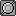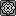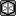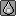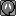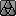Indeed, you can't get Hidden Power Fire 70 BP with 0 Speed IV, unfortunately. The lowest it can be is 2, as you say.

#### Sklez

Thanks for clearing that up. I think I will keep my first HP breeding for a non trickroomer to make things easier.

#### david stone

##### Fast-moving, smart, sexy and alarming.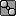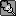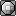You can have HP Fire 64 with 30 Defense, 30 Special Attack, and 0 Speed. This is the highest Hidden Power possible.

#### Aron

This guide= amazingness.
It will be very useful when breeding HP Grass charmanders, and HP Ice eevees.

#### Expert Evan

##### every battle has a smell!It seems the easiest ones to breed for where sp.atk & speed are both at 31 for power(70) are the following in type order:

30/30/30/31/30/31 HP = bug
31/30/30/31/30/31 HP = bug
31/31/31/31/31/31 HP = dark
30/30/31/31/31/31 HP = dragon
30/31/31/31/31/31 HP = dragon
31/30/31/31/31/31 HP = dragon
31/31/30/31/31/31 HP = dragon
30/30/31/31/30/31 HP = ghost
30/31/30/31/30/31 HP = ghost
31/30/31/31/30/31 HP = ghost
31/31/30/31/30/31 HP = ghost
30/30/30/31/31/31 HP = ice
30/31/30/31/31/31 HP = ice
31/30/30/31/31/31 HP = ice
30/31/31/31/30/31 HP = steel
31/31/31/31/30/31 HP = steel

With HP ice being very commonly sought after, I notice there are 3 ways to get a 70 power by focusing on flawless sp.atk/sp.def/speed stats.

#### EconBiscuit

Ok, Gotta say this is really helpful, gonna start attempting to HP Breed...

However instead of doing the Generic 31/30 Speed Breeding I have to do it to TR standards, Do I just subsitute the 31 for 3 and 30 for 2?

#### X-Act

##### np: Biffy Clyro - Shock ShockOk, Gotta say this is really helpful, gonna start attempting to HP Breed...

However instead of doing the Generic 31/30 Speed Breeding I have to do it to TR standards, Do I just subsitute the 31 for 3 and 30 for 2?
Replacing 31 for 3 and 30 for 2 would alter neither the Hidden Power type nor its base power, so yes.

#### EconBiscuit

kk, just making sure, least I've got the basis to help me when I start my project :D

HUGE help tysm

good job

#### HunterVII

lol, still don't quite get it..
how do I breed a modest gengar with HP ice??
ty

#### HunterVII

and also if I bred a gastly how do I check the IV's correctly, thanks!

#### StopSign

thanks for writing this, itll help me breed better

#### Bozo

hidden power FIGHTING
30 speed, special attack and special defense

1.
x/x/x/x/30/x + x/x/x/x/x/x -> x/x/x/x/30/x = 26.5625% = 1:4
2.
x/x/x/x/30/x + x/x/x/x/x/30 -> x/x/x/x/30/30 = 5.95703125% = 1:17
3.
x/x/x/x/30/30 + x/x/x/30/x/x -> x/x/x/30/30/x = 5.95703125% = 1:17, or
x/x/x/x/30/30 + x/x/x/30/x/x -> x/x/x/30/x/30 = 5.95703125% = 1:17
4.
x/x/x/x/30/30 + x/x/x/30/30/x -> x/x/x/30/30/30 = 1.858062744% = 1:54, or
x/x/x/x/30/30 + x/x/x/30/x/30 -> x/x/x/30/30/30 = 1.858062744% = 1:54, or
x/x/x/30/x/30 + x/x/x/30/30/x -> x/x/x/30/30/30 = 1.858062744% = 1:54
5.
x/x/x/30/30/30 + x/x/x/x/30/30 -> x/x/x/30/30/30 = 3.474273682% = 1:29, or
x/x/x/30/30/30 + x/x/x/30/x/30 -> x/x/x/30/30/30 = 3.474273682% = 1:29, or
x/x/x/30/30/30 + x/x/x/30/30/x -> x/x/x/30/30/30 = 3.474273682% = 1:29
6.
x/x/x/30/30/30 + x/x/x/30/30/30 -> x/x/x/30/30/30 = 6.4503479% = 1:16

every approximately 16th baby will now have a chance to have hidden power FIGHTING with base power at least 65, if

A) defense IV is an even number (HP IV and attack IV don ´t matter)

For max base power (70) hidden power FIGHTING, besides getting even defense, you will need the exact IVs of HP, attack and defense to be one of these:
31/30, 27/26, 23/22, 19/18, 15/14, 11/10, 7/6, 3/2
hp fighting can also be obtained with E/E/O/E/E/E.

i'm pretty sure this is the only combination which isnt mentioned explicitly in the first post. (except for some that were intentionally left out - eg the slow hp ice spread etc).

(i'm not really a nerd. i was just making a table of all the even/odd combinations and the hp types they produce. nothing nerdy at all.....)

#### X-Act

##### np: Biffy Clyro - Shock ShockYou're right. You can have 30/30/31/30/30/30, 31/31/30/30/30/30, 31/30/30/30/30/30, 30/31/30/30/30/30 or 30/30/30/30/30/30 to get HP Fighting 70.

#### Philip-B

I just want to thank you guys for this. you don't know how much this has helped me with HP.It helped a lot. well, now you do. Kudos, anyway

#### Bungalow Bill

Bloody brilliant, this helps me out a lot.